Search by Topic

Resources tagged with Engineering similar to Pumping the Power:

Filter by: Content type:
Age range:
Challenge level:

There are 56 results

Broad Topics > Applications > EngineeringPumping the Power

Age 16 to 18 Challenge Level:

What is an AC voltage? How much power does an AC power source supply?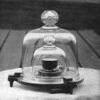New Units for Old

Age 16 to 18 Challenge Level:

Can you match up the entries from this table of units?Powerfully Fast

Age 16 to 18 Challenge Level:

Explore the power of aeroplanes, spaceships and horses.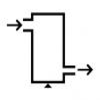Keep Your Momentum Going

Age 16 to 18 Challenge Level:

A look at a fluid mechanics technique called the Steady Flow Momentum Equation.Stemnrich - Technology

Age 11 to 16 Challenge Level:

This is the technology section of stemNRICH - Core.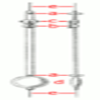The Not-so-simple Pendulum 2

Age 16 to 18 Challenge Level:

Things are roughened up and friction is now added to the approximate simple pendulumMoving Stonehenge

Age 16 to 18 Challenge Level:

A look at the fluid mechanics questions that are raised by the Stonehenge 'bluestones'.Go Spaceship Go

Age 16 to 18 Challenge Level:

Show that even a very powerful spaceship would eventually run out of overtaking powerThe Not-so-simple Pendulum 1

Age 16 to 18 Challenge Level:

See how the motion of the simple pendulum is not-so-simple after all.Battery Modelling

Age 16 to 18 Challenge Level:

Find out how to model a battery mathematicallyMach Attack

Age 16 to 18 Challenge Level:

Have you got the Mach knack? Discover the mathematics behind exceeding the sound barrier.Ramping it Up

Age 16 to 18 Challenge Level:

Look at the calculus behind the simple act of a car going over a step.The Power of Dimensional Analysis

Age 14 to 18

An introduction to a useful tool to check the validity of an equation.Core Scientific Mathematics

Age 14 to 18 Challenge Level:

This is the area of the advanced stemNRICH site devoted to the core applied mathematics underlying the sciences.Maths in the Undergraduate Physical Sciences

Age 16 to 18

An article about the kind of maths a first year undergraduate in physics, engineering and other physical sciences courses might encounter. The aim is to highlight the link between particular maths. . . .Making More Tracks

Age 16 to 18 Challenge Level:

Given the equation for the path followed by the back wheel of a bike, can you solve to find the equation followed by the front wheel?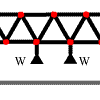More Bridge Building

Age 16 to 18 Challenge Level:

Which parts of these framework bridges are in tension and which parts are in compression?Engnrich

Age 16 to 18 Challenge Level:

engNRICH is the area of the stemNRICH Advanced site devoted to the mathematics underlying the study of engineeringPhysnrich

Age 14 to 18 Challenge Level:

PhysNRICH is the area of the StemNRICH site devoted to the mathematics underlying the study of physicsAC/DC Circuits

Age 16 to 18

This article, including exercises, gives a thorough grounding in the topic of AC/DC circuits.Impedance Can Be Complex!

Age 16 to 18 Challenge Level:

Put your complex numbers and calculus to the test with this impedance calculation.Approximately Certain

Age 14 to 18 Challenge Level:

Estimate these curious quantities sufficiently accurately that you can rank them in order of sizeThe Wheatstone Bridge

Age 16 to 18 Challenge Level:

Explore the mathematics behind the famous Wheatstone Bridge circuit.Dangerous Driver?

Age 16 to 18 Challenge Level:

Was it possible that this dangerous driving penalty was issued in error?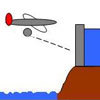Dam Busters 2

Age 16 to 18 Challenge Level:

Can you work out which of the equations models a bouncing bomb? Will you be able to hit the target?Model Solutions

Age 16 to 18 Challenge Level:

How do these modelling assumption affect the solutions?Turbo Turbines

Age 16 to 18 Challenge Level:

A look at power generation using wind turbines.Building up Friction

Age 16 to 18 Challenge Level:

A series of activities to build up intuition on the mathematics of friction.Overarch 1

Age 16 to 18 Short Challenge Level:

This short question asks if you can work out the most precarious way to balance four tiles.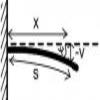Beam Me Up

Age 16 to 18 Challenge Level:

A preview of some of the beam deflection mechanics you will look at in the first year of an engineering degree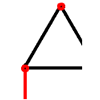Bridge Builder

Age 16 to 18 Challenge Level:

In this short problem we investigate the tensions and compressions in a framework made from springs and ropes.Dam Busters 1

Age 16 to 18 Challenge Level:

At what positions and speeds can the bomb be dropped to destroy the dam?Stonehenge Is Going Nowhere

Age 14 to 16 Challenge Level:

In an extension to the Stonehenge problem, consider the mechanical possibilities for an arrangement of frictional rollers.Euler's Buckling Formula

Age 16 to 18 Challenge Level:

Derive Euler's buckling formula from first principles.Not Another NAND!

Age 14 to 18 Challenge Level:

Prove that you can make any type of logic gate using just NAND gates.Differential Electricity

Age 16 to 18 Challenge Level:

As a capacitor discharges, its charge changes continuously. Find the differential equation governing this variation.A Circuit Problem

Age 16 to 18 Short Challenge Level:

Explore the voltages and currents in this interesting circuit configuration.Maximum Flow

Age 16 to 18 Challenge Level:

Given the graph of a supply network and the maximum capacity for flow in each section find the maximum flow across the network.Stonehenge

Age 16 to 18 Challenge Level:

Explain why, when moving heavy objects on rollers, the object moves twice as fast as the rollers. Try a similar experiment yourself.Overarch 2

Age 16 to 18 Challenge Level:

Bricks are 20cm long and 10cm high. How high could an arch be built without mortar on a flat horizontal surface, to overhang by 1 metre? How big an overhang is it possible to make like this?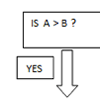Mystery Procedure

Age 14 to 16 Challenge Level:

Can you work out what this procedure is doing?Procedure Solver

Age 16 to 18 Challenge Level:

Can you think like a computer and work out what this flow diagram does?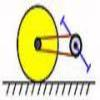Who Is an Engineer? A Quick Perspective After One Year of a University Engineering Course

Age 16 to 18

Doug has just finished the first year of his undergraduate engineering course at Cambridge University. Here he gives his perspectives on engineering.